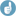## R inferno (without rant) [Software]

Dear Helmut,

» ifelse (x1 < x2, »   cat(x1, "less than", x2, "\n"), »   cat(x1, "at least", x2, "\n"))» » 12 less than 13 » Error in ifelse(x1 < x2, cat(x1, "less than", x2, "\n"), cat(x1, "at least",  : »   replacement has length zero» In addition: Warning message:» In rep(yes, length.out = length(ans)) : »   'x' is NULL so the result will be NULL
»    What the f…k?

Not only SAS is a beast.

ifelse() is a (vectorized) function for conditional replacement / selection of values, not for conditional doing sumfink like output with cat().

From the man page of ifelse():
Usage
ifelse(test, yes, no)

Arguments
test  an object which can be coerced to logical mode.
yes   return values for true elements of test.
no    return values for false elements of test.

What is the return value of cat()?:
x <- cat("sumfink\n") x

Gives NULL.

From the man page of cat():
Value
None (invisible NULL).

All the f#+~ing behaviour as described and documented. No reason to rant.
For some inferno <- TRUE see Patrick Burns "R Inferno" Circle 3.2 and 8.2.7

If you really need a vectorized solution (if x1, x2 are vectors) I suggest the following:
cat(ifelse(x1 < x2,            paste0(x1, " less than ", x2),            paste0(x1, " at least ", x2 )           ), sep="\n")

Try it with
x1 <- c(12, 13) x2 <- c(13, 12)

BTW: Works of course also if x1 and x2 are scalars as in your example.

Regards,

DetlewIng. Helmut Schütz